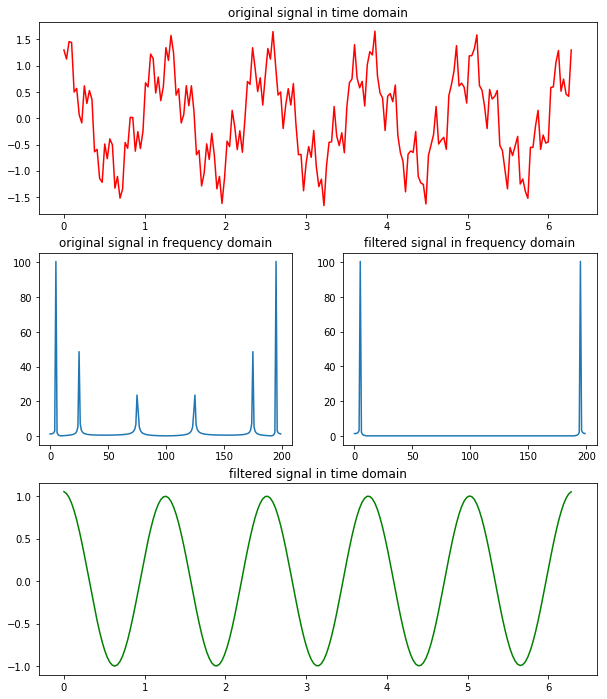## 1. 傅里叶级数 Fourier Series (FS)

$f(t) = \dfrac{a_0}2 + \sum\limits_{n=1}^\infty\left(a_n\cos\dfrac{2\pi n}Tt + b_n\sin\dfrac{2\pi n}Tt\right) \tag{1}$

$a_n = \dfrac2T\int_{t_0}^{t_0+T}f(t)\cos\dfrac{2\pi n}Tt\mathrm{d}t, n=0, 1, 2, \cdots \tag{2}$

$b_n = \dfrac2T\int_{t_0}^{t_0+T}f(t)\sin\dfrac{2\pi n}Tt\mathrm{d}t, n=1, 2, \cdots \tag{3}$

\begin{aligned} \cos\theta &= \dfrac12\left(\mathrm{e}^{i\theta}+\mathrm{e}^{-i\theta}\right)\\ \sin\theta &= -\dfrac i2\left(\mathrm{e}^{i\theta}-\mathrm{e}^{-i\theta}\right) \end{aligned} \tag{4}

$f(t) = \dfrac{a_0}2 + \sum\limits_{n=1}^\infty\left(\dfrac{a_n-ib_n}2\mathrm{e}^{i\frac{2\pi n}Tt} + \dfrac{a_n+ib_n}2\mathrm{e}^{-i\frac{2\pi n}Tt}\right) \tag{5}$

$c_n = \begin{cases} \dfrac{a_n - ib_n}2 &\text{n>0}\\ \dfrac{a_0}2 &\text{n=0}\\ \dfrac{a_{|n|} + ib_{|n|}}2 &\text{n<0} \end{cases} \tag{6}$

$f(t) = \sum\limits_{n=-\infty}^{+\infty}c_n\mathrm{e}^{i\frac{2\pi n}Tt}\tag{7}$

$c_n = \dfrac1T\int_{t_0}^{t_0+T}f(t)\mathrm{e}^{-i\frac{2\pi n}Tt}\mathrm{d}t, \qquad n=0, \pm1 \pm2,\cdots\tag{8}$

## 2. 傅里叶变换 Fourier Transform (FT)

$f(t) = \sum\limits_{n=-\infty}^{+\infty}c_n\mathrm{e}^{i\frac{2\pi n}Tt}\tag{9}$

$c_n = \dfrac1T\int_{-T/2}^{T/2}f(t)\mathrm{e}^{-i\frac{2\pi n}Tt}\mathrm{d}t, \qquad n=0, \pm1 \pm2,\cdots\tag{10}$

$\Delta\omega=\dfrac{2\pi}T\tag{11}$

$\hat{f}(n\Delta\omega)=Tc_n=\int_{-T/2}^{T/2}f(t)\mathrm{e}^{-in\Delta\omega t}\mathrm{d}t, \qquad n=0, \pm1, \pm2, \cdots\tag{12}$

$c_n=\dfrac{\hat{f}(n\Delta\omega)}T=\dfrac{\Delta\omega}{2\pi}\hat{f}(n\Delta\omega)\tag{13}$

\begin{aligned} f(t) &= \sum\limits_{n=-\infty}^{+\infty}c_n\mathrm{e}^{i\frac{2\pi n}Tt} \\ &= \dfrac1{2\pi} \sum\limits_{n=-\infty}^{+\infty}\hat{f}(n\Delta\omega)\mathrm{e}^{in\Delta\omega t}\Delta\omega \end{aligned} \tag{14}

$T\to\infty$ 时，$n\Delta\omega\to\omega$$\Delta\omega\to\mathrm{d}\omega$， (14) 中的求和变为积分

$f(t) = \dfrac1{2\pi}\int_{-\infty}^{+\infty}\hat{f}(\omega)\mathrm{e}^{i\omega t}\mathrm{d}\omega\tag{15}$

$\hat{f}(\omega)=\int_{-\infty}^{+\infty}f(t)\mathrm{e}^{-i\omega t}\mathrm{d}t\tag{16}$

(16) 称为傅里叶变换，记作 $\hat{f}(\omega) = \mathcal F[f](t)$；(15) 称为傅里叶变换的逆变换，记作 $f(t) = \mathcal F^{-1}[\hat{f}](\omega)$。在信号分析中，$f(t)$ 称为信号的时域表示，$\hat{f}(\omega)$ 称为信号的频域表示。

$\hat{f}(\nu) =\mathcal Ff(t)= \int_{-\infty}^{+\infty}f(t)\mathrm{e}^{-i2\pi\nu t}\mathrm{d}t\tag{17}$

$f(t) =\mathcal F^{-1}\hat{f}(\nu) = \int_{-\infty}^{+\infty}\hat{f}(\xi)\mathrm{e}^{i2\pi\nu t}\mathrm{d}\nu\tag{18}$

## 3. 离散时间傅里叶变换 Discrete-time Fourier Transform (DTFT)

$f_n = f(n\tau), \qquad n=0, \pm1, \pm2, \cdots\tag{19}$

$\tau$ 是采样的时间间隔。傅里叶变换只能作用在连续函数上，为此我们引入

\begin{aligned} f_s(t) &= f(t)\sum\limits_{n=-\infty}^{+\infty}\delta(t-n\tau)\\ &=\sum\limits_{n=-\infty}^{+\infty}f(t)\delta(t-n\tau)\\ &=\sum\limits_{n=-\infty}^{+\infty}f_n\delta(t-n\tau) \end{aligned} \tag{20}

$\delta(x) = \begin{cases} +\infty, &x=0\\ 0, &x\neq0 \end{cases} \tag{21}$

$f_s(t)$ 作傅里叶变换

\begin{aligned} \hat{f}_s(\omega)&=\int_{-\infty}^{+\infty}f_s(t)\mathrm{e}^{-i\omega t}\mathrm{d}t\\ &=\int_{-\infty}^{+\infty}\sum\limits_{n=-\infty}^{+\infty}f(t)\delta(t-n\tau)\mathrm{e}^{-i\omega t}\mathrm{d}t\\ &=\sum\limits_{n=-\infty}^{+\infty}\int_{-\infty}^{+\infty}\delta(t-n\tau)f(t)\mathrm{e}^{-i\omega t}\mathrm{d}t\\ &=\sum\limits_{n=-\infty}^{+\infty}f_n\mathrm{e}^{-i\omega n\tau} \end{aligned} \tag{22}

$\hat{f}(\omega)$$[-\frac{\omega_s}2, \frac{\omega_s}2]$ 上展开为傅里叶级数

$\hat{f}(\omega) = \sum\limits_{m=-\infty}^{+\infty}c_m\mathrm e^{i\frac{2\pi m}{\omega_s}\omega}\tag{23}$

$c_m = \frac 1{\omega_s}\int_{-\omega_s/2}^{\omega_s/2}\hat{f}(\omega)\mathrm e^{-i\frac{2\pi m}{\omega_s}\omega}\mathrm d\omega\tag{24}$

\begin{aligned} c_m &= \frac 1{\omega_s}\int_{-\infty}^{+\infty}\hat{f}(\omega)\mathrm e^{-i\frac{2\pi m}{\omega_s}\omega}\mathrm d\omega\\ &=\frac{2\pi}{\omega_s}f(-\frac{2\pi}{\omega_s}m)\\ &=\tau f(-m\tau)\\ &=\tau f_{-m} \end{aligned} \tag{25}

\begin{aligned} \hat{f}(\omega) &= \sum\limits_{m=-\infty}^{+\infty}\tau f_{-m}\mathrm e^{i\frac{2\pi m}{\omega_s} \omega}\\ &= \sum\limits_{n=-\infty}^{+\infty}\tau f_{n}\mathrm e^{-i\frac{2\pi n}{\omega_s}\omega}\\ &= \tau\sum\limits_{n=-\infty}^{+\infty}f_n\mathrm{e}^{-i\omega n\tau }\\ &= \tau\hat{f}_s(\omega) \end{aligned} \tag{26}

\begin{aligned} \hat{f}_s(\omega) &= \sum\limits_{n=-\infty}^{+\infty}f_n \mathrm{e}^{-i\omega n\tau } \\ &= \sum\limits_{n=-\infty}^{+\infty}f_n\mathrm{e}^{-i2\pi n\frac \omega{\omega_s}} \end{aligned} \tag{27}

## 4. 离散傅里叶变换 Discrete Fourier Transform (DFT)

$\hat{f}_k = \sum\limits_{n=0}^{N-1}f_n\mathrm{e}^{-i\frac{2\pi}Nnk}, \qquad k=0, 1, \cdots, N-1\tag{28}$

$\left[ \begin{matrix} \hat{f}_0\\ \hat{f}_1\\ \hat{f}_2\\ \vdots\\ \hat{f}_{N-1} \end{matrix} \right] =\left[ \begin{matrix} 1 & 1 & 1 & \cdots & 1\\ 1 & \varphi & \varphi^2 & \cdots & \varphi^{ N-1}\\ 1 & \varphi^2 &\varphi^4 & \cdots & \varphi^{2(N-1)}\\ \vdots & \vdots & \vdots & \ddots & \vdots\\ 1 & \varphi^{N-1} & \varphi^{2(N-1)} & \cdots & \varphi^{(N-1)^2} \end{matrix} \right] \left[ \begin{matrix} f_0\\ f_1\\ f_2\\ \vdots\\ f_{N-1} \end{matrix} \right] \tag{29}$

$f_n = \frac{1}{N}\sum\limits_{k=0}^{N-1}\hat{f}_k\mathrm e^{i\frac{2\pi}{N}nk}, \qquad n=0, 1, \cdots, N-1 \tag{30}$

## 5. 快速傅里叶变换 Fast Fourier Transform (FFT)